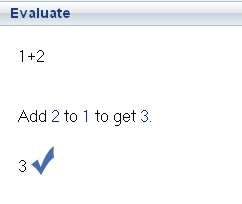Having problems with calculus, algebra, geometry or vectors in your Math homework? Or probably (sin(y) – tan (x)) / (sec2(x) – 1) are giving you headache? Well, if you get stuck doing your mathematics homework or trying to resolve some math problems for your children, there is a free Mathematics tutor, Mathway, which can assist you.Mathway is a good helper for students, parents and even teachers to solve mathematics problems. Unlike a mathematic calculator, Mathway will resolve users’ mathematic problems based on users’ problem statements. For instance, if you want to get the answer of 1+2, then you can select the subject (Basic Math in this case) and thence enter the statement “Add 1 to 2” or “1+2”. The site is smart enough to identify the problem and get you the correct answer.

There are various categories, namely “Basic Maths”, “Pre-Algebra”, “Algebra”, “Trigonometry”, “Precalculus”, and “Calculus” for users to select. Users need to choose the relevant category in order to get the respective symbols which are commonly used to enter the “problem statement”. For instance, if users click on “Trigonometry”, a set of useful toolbar buttons showing related math symbols and expressions such as log, sin, cos, tan, csc, cot, etc will appear. With these symbols, users can enter their problem statements much easier and faster. Those who need to look up the meaning of mathematics terminology can browse the glossary list in Mathway. Besides, users can also use the step-by-step graph plotting option to create graphs. Users can embed the graphs to their blogs or websites once they are completed.

Mathway is a free service. Get this step-by-step arithmetic problem solver to assist you with your mathematical woes or simply to make solving problems easier and more fun.